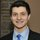# Resetting Cumulative Sums By Condition

Suppose you have a table period_end that indicates if a user is paying at the end of each period, like so:

```period   paying
1        1
2        1
3        1
4        0
5        1
```

You want to calculate the number of consecutive periods paying at time x. Typically, you would use a sum window function. The problem is that the window function resumes where it leaves off, like so:

```period  paying  window_sum
1       1         1
2       1         2
3       1         3
4       0         3
5       1         4
```

To get the sum of consecutive paying periods, we actually want a result that looks like this:

```period  paying  consec_periods
1       1           1
2       1           2
3       1           3
4       0           0
5       1           1
```

To get the above, we first need to identify groups of consecutive periods where paying = 1 or 0. We can do this with the lag function:

```with x as (
select
period
, paying
, case when paying = lag(paying,1) over (order by period) then 0 else 1
end as chg
from period_end
)

select
period
, paying
, sum(chg) over (order by period) as grp
from x
```

The above outputs the following:

```period  paying  grp
1       1      1
2       1      1
3       1      1
4       0      2
5       1      3
```

Now, we can compute a window sum of paying over grp ordered by period to get our desired output (see second table above):

```with x as (
select
period
, paying
, case when paying = lag(paying,1) over (order by period) then 0 else 1
end as chg
from period_end
)
,

y as (
select
period
, paying
, sum(chg) over (order by period) as grp
from x
)

select
period
, paying
, sum(paying) over (partition by grp order by period) as consec_periods
from y
```

Your period end table will likely also include a user_id column. Include the user_id in the partition clause of the last part of the query and you're good to go!

2replies Oldest first
• Oldest first